Next: The Static Load Balancing Up: Load Balancing for Unstructured Previous: Introduction

# Graph Theory Notation

Much of the load balancing problem can be described using terminology from graph theory. In fact some of these problems had already been studied in graph theory before they appeared in the context of parallel computing.

A graph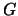has two key components:

• The vertex set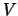, which is just a list of indices;
• The edge set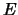. Each edge consists of two vertices.
The number of vertices and edges of a graph are denoted by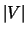and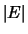. The number of edges connected to a vertex is called the degree of the vertex. The degree of the graph is the largest degree of all of its vertices.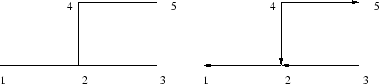A graph can be directed, in which case there is a beginning and an end vertex (also called the head and the tail, respectively) for each edge. A graph can also be undirected. For example, Figure 1 illustrates an undirected graph (left) and a directed graph (right). The undirected graph has 5 vertices,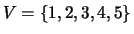, and 4 edges,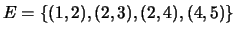. The number of vertices and edges are therefore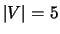and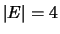respectively. The directed graph also has 5 vertices and 4 edges, although each edge has a direction associated. For instance, the edge between vertex 4 and 2 has vertex 4 as the head and vertex 2 as the tail. All graphs in this paper are undirected, unless specified otherwise.

If two vertices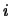and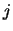form an edge, this will be denoted as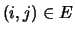, or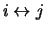. A graph is connected, if for any two verticesand, there exists a path connecting them. Here a path is defined as a list of vertices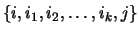such that any two subsequent vertices in this list form an edge.

The distance between two vertices of a graph is defined as the minimum number of edges among all paths connecting these two vertices. For the undirected graph in Figure 1, the distance between vertex 1 and vertex 5 is 3. This is different to the physical distance between the two points in the Euclidean space.Next: The Static Load Balancing Up: Load Balancing for Unstructured Previous: Introduction

2000-03-21